# Class 9 NCERT Solutions – Chapter 6 Lines And Angles – Exercise 6.3

• Last Updated : 11 Dec, 2020

### Question1: In Figure, sides QP and RQ of ΔPQR are produced to points S and T respectively. If ∠SPR = 135° and ∠PQT = 110°, find ∠PRQ.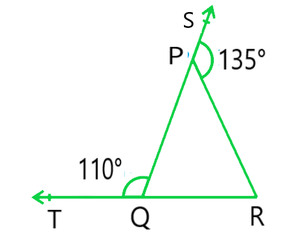Solution:

Given: ∠TQP = 110°, ∠SPR = 135°

TQR is a Straight line as we can see in the figure

As we have studied in this chapter, TQP and PQR will form a linear pair

⇒ ∠TQP + ∠PQR = 180°  ———-(i)

Putting the value of ∠TQP = 110° in Equation (i) we get,

⇒ 110° + ∠PQR = 180°

⇒ ∠PQR = 70°

Consider the ΔPQR,

Here, the side QP is extended to S and so, SPR forms the exterior angle.

Thus, ∠SPR (∠SPR = 135°) is equal to the sum of interior opposite angles. (Triangle property)

Or, ∠PQR + ∠PRQ = 135° ———(ii)

Now, putting the value of PQR = 70° in equation (ii) we get,

∠PRQ = 135° – 70°

Hence, ∠PRQ = 65°

### Question 2: In Figure, ∠X = 62°, ∠ XYZ = 54°. If YO and ZO are the bisectors of XYZ and XZY respectively of Δ XYZ, find OZY and YOZ.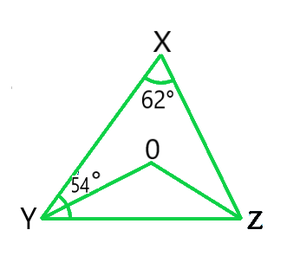Solution:

Given: ∠X = 62°, ∠XYZ = 54°

As we have studied in this chapter,

We know that the sum of the interior angles of the triangle is 180°.

So, ∠X +∠XYZ +∠XZY = 180°

Putting the values as given in the question we get,

62°+54° + ∠XZY = 180°

Or, ∠XZY = 64°

Now, we know that ZO is the bisector so,

∠OZY = ½ XZY

∴ ∠OZY = 32°

Similarly, YO is a bisector and so,

∠OYZ = ½ XYZ

Or, ∠OYZ = 27° (As XYZ = 54°)

Now, as the sum of the interior angles of the triangle,

∠OZY +∠OYZ +O = 180°

Putting their respective values, we get,

∠O = 180°-32°-27°

Hence, ∠O = 121°

### Question 3: In Figure, if AB || DE, ∠BAC = 35° and ∠CDE = 53°, find ∠DCE.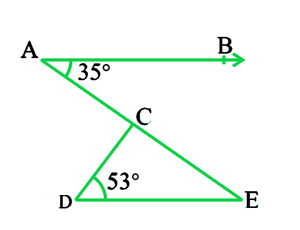Solution:

Given: AB || DE, ∠BAC = 35° and ∠CDE = 53°

Since, we know that AE is a transversal of AB and DE

Here, BAC and AED are alternate interior angles.

Hence, ∠BAC = ∠AED

∠BAC = 35° (Given)

∠AED = 35°

Now consider the triangle CDE. We know that the sum of the interior angles of a triangle is 180°.

∴ ∠DCE + ∠CED + ∠CDE = 180°

Putting the values, we get

∠DCE + 35° + 53° = 180°

Hence, ∠DCE = 92°

### Question 4: In Figure, if lines PQ and RS intersect at point T, such that ∠PRT = 40°, ∠RPT = 95° and ∠TSQ = 75°, find ∠SQT.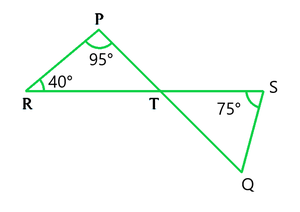Solution:

Given: ∠PRT = 40°, ∠RPT = 95° and ∠TSQ = 75°

In △PRT.

∠PRT +∠RPT + ∠PTR = 180° (The Sum of all the angles of Triangle is 180°)

⇒ ∠PTR = 45°

Now ∠PTR will be equal to STQ as they are vertically opposite angles.

⇒ ∠PTR = ∠STQ = 45°

Again, in triangle STQ,

⇒ ∠TSQ +∠PTR + ∠SQT = 180°  (The Sum of all the angles of Triangle is 180°)

Solving this we get,

⇒ ∠SQT = 60°

### Question 5: In Figure, if PQ ⊥ PS, PQ || SR, ∠SQR = 28° and ∠QRT = 65°, then find the values of x and y.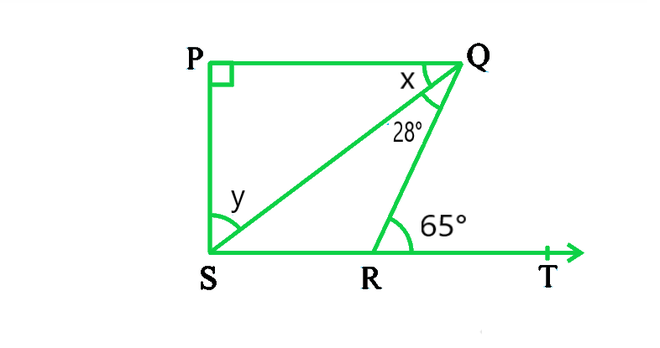Solution:

Given: PQ ⊥ PS, PQ || SR, ∠SQR = 28° and ∠QRT = 65°

x + SQR = QRT (As they are alternate angles since QR is transversal)

Now, Putting the value of ∠SQR = 28° and ∠QRT = 65°

⇒ x + 28° = 65°

∴ x = 37°

It is also known that alternate interior angles are same and

⇒ QSR = x = 37°

Also,

⇒ QRS + QRT = 180° (As they form a Linear pair)

Putting the value of ∠QRT = 65° we get,

⇒ QRS + 65° = 180°

⇒ QRS = 115°

As we know that the sum of the angles in a quadrilateral is 360°.

⇒ P + Q + R + S = 360°

Putting their respective values, we get,

⇒ S = 360° – 90° – 65° – 115° = 900

In Δ SPQ

⇒ ∠SPQ + x + y = 1800

⇒ 900 + 370 + y = 1800

⇒ y = 1800 – 1270 = 530

Hence, y = 53°

### Question 6: In Figure, the side QR of ΔPQR is produced to a point S. If the bisectors of ∠PQR and ∠PRS meet at point T, then prove that ∠QTR = ½ ∠QPR.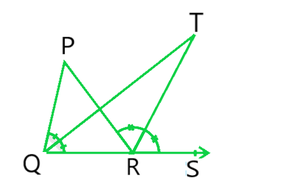Solution:

Given: T is the bisector of ∠PQR and ∠PRS,

To Prove: ∠QTR = ½ ∠QPR

Proof:

Consider the ΔPQR.

∠PRS is an exterior angle.

∠QPR and ∠PQR are interior angles.

⇒ ∠PRS = ∠QPR + ∠PQR (According to triangle property)

⇒ ∠PRS – ∠PQR = ∠QPR  ————(i)

Now, consider the ΔQRT,

∠TRS = ∠TQR + ∠QTR (Since exterior angle are equal)

⇒ ∠QTR = ∠TRS – ∠TQR

We know that QT and RT bisect ∠PQR and ∠PRS respectively.

So, ∠PRS = 2 ∠TRS and ∠PQR = 2∠TQR

Now,

⇒ ∠QTR = ½ ∠PRS – ½ ∠PQR

⇒ ∠QTR = ½ ∠(PRS – PQR)

From equation (i) we know that ∠PRS – ∠PQR = ∠QPR,

⇒ ∠QTR = ½ ∠QPR

Hence, Proved.

My Personal Notes arrow_drop_up